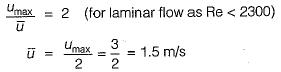Courses

# Test: Viscous Flow - 1

## 10 Questions MCQ Test Topicwise Question Bank for Mechanical Engineering | Test: Viscous Flow - 1

Description
This mock test of Test: Viscous Flow - 1 for Mechanical Engineering helps you for every Mechanical Engineering entrance exam. This contains 10 Multiple Choice Questions for Mechanical Engineering Test: Viscous Flow - 1 (mcq) to study with solutions a complete question bank. The solved questions answers in this Test: Viscous Flow - 1 quiz give you a good mix of easy questions and tough questions. Mechanical Engineering students definitely take this Test: Viscous Flow - 1 exercise for a better result in the exam. You can find other Test: Viscous Flow - 1 extra questions, long questions & short questions for Mechanical Engineering on EduRev as well by searching above.
QUESTION: 1

Solution:
QUESTION: 2

### An oil of kinematic viscosity 0.5 stokes flows through a pipe of 4 cm diameter. The flow is critical at a velocity of about

Solution: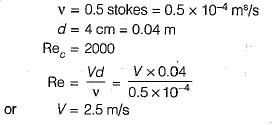QUESTION: 3

### In flow through a pipe, the transition from laminar to turbulent flow does not depend on

Solution:

Transition depends on Reynolds number Reynolds number depends on velocity, diameter, dynamic/kinematic viscosity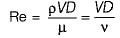QUESTION: 4

The equations of motion for a viscous fluid are known as

Solution:
QUESTION: 5

In the Navier-Stokes equations, the forces considered are

Solution:
QUESTION: 6

Navier-Stoke’s equation represents the conservation of

Solution:

Navier-stokes equations represent the conservation of momentum, are equation of motion for viscous fluid and consider combination of gravity, pressure and viscous forces.

QUESTION: 7

For laminar flow between two fixed parallel plates, the flow velocity

Solution: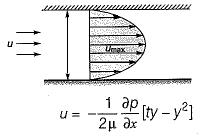QUESTION: 8

The maximum velocity of a one-dimensional incompressible flow between two fixed parallel plates is 5 m/s. The mean velocity (in m/s) of the flow is

Solution: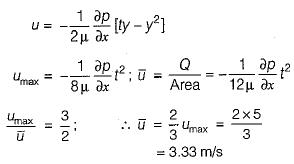QUESTION: 9

The shear stress between two fixed parallel plates with a laminar flow between them

Solution: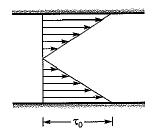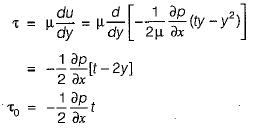QUESTION: 10

Velocity for flow through a pipe, measured at the center is found to be 3 m/s. If the Reynolds number is around 1000, what is the average flow velocity in the pipe?

Solution: